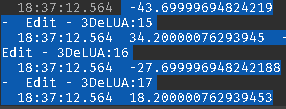# Why am I getting this error? (HELP!)

This is the code:

``````local Max_X, Max_Y = position.X+(-size.X/2), position.Y+(size.Y/2)
local Min_X, Min_Y = position.X+(size.X/2), position.Y+(-size.Y/2)

local X1 = math.random(Min_X,Max_X)
local Y1 = math.random(Min_Y,Max_Y)

local X2 = math.random(Min_X,Max_X)
local Y2 = math.random(Min_Y,Max_Y)
``````

And it is giving these values:But it returns the error when I do all the randoms:Anyone know why? All the intervals are valid, I’ve even used print to receive the values that are returning errors. When I do a manual math.Random() with the values, it works. But in the module, it gives any error.

seems like your Min_X might be higher than your Max_X. I cannot tell what values you are getting relate to what variables, but the first argument must be less than the second argument in math.random

Just use Random.new():NextInteger(), it doesnt have that problem.

1 Like
``````local Max_X, Max_Y = position.X+(-size.X/2), position.Y+(size.Y/2)
local Min_X, Min_Y = position.X+(size.X/2), position.Y+(-size.Y/2)
``````

The minus sign is wrong. It should be:

``````local Max_X, Max_Y = position.X+(size.X/2), position.Y+(size.Y/2)
local Min_X, Min_Y = position.X+(-size.X/2), position.Y+(-size.Y/2)

``````

It’s because the values were negative. This completely fixed it.

This topic was automatically closed 14 days after the last reply. New replies are no longer allowed.